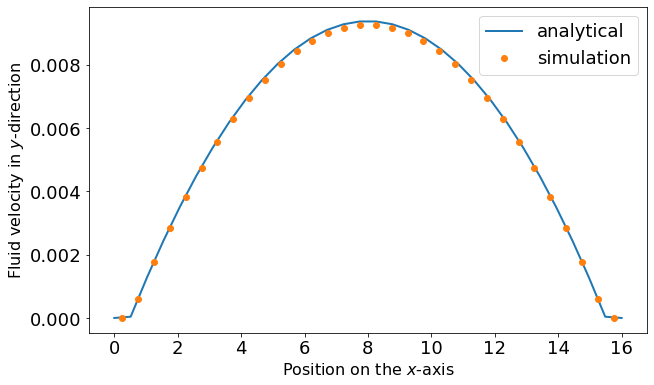# Poiseuille flow in ESPResSo¶

Poiseuille flow is the flow through a pipe or (in our case) a slit under a homogeneous force density, e.g. gravity. In the limit of small Reynolds numbers, the flow can be described with the Stokes equation. We assume the slit being infinitely extended in $y$ and $z$ direction and a force density $f_y$ on the fluid in $y$ direction. No slip-boundary conditions (i.e. $\vec{u}=0$) are located at $x = \pm h/2$. Assuming invariance in $y$ and $z$ direction and a steady state, the Stokes equation is simplified to:

\begin{equation} \mu \partial_x^2 u_y = f_y \end{equation}

where $f_y$ denotes the force density and $\mu$ the dynamic viscosity. This can be integrated twice and the integration constants are chosen so that $u_y=0$ at $x = \pm h/2$ to obtain the solution to the planar Poiseuille flow :

\begin{equation} u_y(x) = \frac{f_y}{2\mu} \left(h^2/4-x^2\right) \end{equation}

We will simulate a planar Poiseuille flow using a square box, two walls with normal vectors $\left(\pm 1, 0, 0 \right)$, and an external force density applied to every node.

## 1. Setting up the system¶

In :
import logging
import sys

%matplotlib inline
import matplotlib.pyplot as plt

In :
plt.rcParams.update({'font.size': 18})
import numpy as np
import tqdm

import espressomd
import espressomd.lb
import espressomd.lbboundaries
import espressomd.shapes

logging.basicConfig(level=logging.INFO, stream=sys.stdout)

espressomd.assert_features(['LB_BOUNDARIES_GPU'])

# System constants
BOX_L = 16.0
TIME_STEP = 0.01

system = espressomd.System(box_l=[BOX_L] * 3)
system.time_step = TIME_STEP
system.cell_system.skin = 0.4


### 1.1 Setting up the lattice-Boltzmann fluid¶

We will now create a lattice-Boltzmann fluid confined between two walls.

In :
# LB parameters
AGRID = 0.5
VISCOSITY = 2.0
FORCE_DENSITY = [0.0, 0.001, 0.0]
DENSITY = 1.5

# LB boundary parameters
WALL_OFFSET = AGRID


Create a lattice-Boltzmann actor and append it to the list of system actors. Use the GPU implementation of LB.

You can refer to section setting up a LB fluid in the user guide.

In :
logging.info("Setup LB fluid.")
lbf = espressomd.lb.LBFluidGPU(agrid=AGRID, dens=DENSITY, visc=VISCOSITY, tau=TIME_STEP,
ext_force_density=FORCE_DENSITY)

INFO:root:Setup LB fluid.


Create a LB boundary and append it to the list of system LB boundaries.

You can refer to section using shapes as lattice-Boltzmann boundary in the user guide.

In :
logging.info("Setup LB boundaries.")
top_wall = espressomd.shapes.Wall(normal=[1, 0, 0], dist=WALL_OFFSET)
bottom_wall = espressomd.shapes.Wall(normal=[-1, 0, 0], dist=-(BOX_L - WALL_OFFSET))

top_boundary = espressomd.lbboundaries.LBBoundary(shape=top_wall)
bottom_boundary = espressomd.lbboundaries.LBBoundary(shape=bottom_wall)


INFO:root:Setup LB boundaries.

Out:
<espressomd.lbboundaries.LBBoundary at 0x7f67f0020cc0>

## 2. Simulation¶

We will now simulate the fluid flow until we reach the steady state.

In :
logging.info("Iterate until the flow profile converges (5000 LB updates).")
for _ in tqdm.trange(20):
system.integrator.run(5000 // 20)

INFO:root:Iterate until the flow profile converges (5000 LB updates).

100%|██████████| 20/20 [00:02<00:00,  7.94it/s]


## 3. Data analysis¶

We can now extract the flow profile and compare it to the analytical solution for the planar Poiseuille flow.

In :
logging.info("Extract fluid velocities along the x-axis")

fluid_positions = (np.arange(lbf.shape) + 0.5) * AGRID
# get all velocities as Numpy array and extract y components only
fluid_velocities = (lbf[:,:,:].velocity)[:,:,:,1]
# average velocities in y and z directions (perpendicular to the walls)
fluid_velocities = np.average(fluid_velocities, axis=(1,2))

def poiseuille_flow(x, force_density, dynamic_viscosity, height):
return force_density / (2 * dynamic_viscosity) * (height**2 / 4 - x**2)

# Note that the LB viscosity is not the dynamic viscosity but the
# kinematic viscosity (mu=LB_viscosity * density)
x_values = np.linspace(0.0, BOX_L, lbf.shape)
HEIGHT = BOX_L - 2.0 * AGRID
# analytical curve
y_values = poiseuille_flow(x_values - (HEIGHT / 2 + AGRID), FORCE_DENSITY,
VISCOSITY * DENSITY, HEIGHT)
# velocity is zero inside the walls
y_values[np.nonzero(x_values < WALL_OFFSET)] = 0.0
y_values[np.nonzero(x_values > BOX_L - WALL_OFFSET)] = 0.0

fig1 = plt.figure(figsize=(10, 6))
plt.plot(x_values, y_values, '-', linewidth=2, label='analytical')
plt.plot(fluid_positions, fluid_velocities, 'o', label='simulation')
plt.xlabel('Position on the $x$-axis', fontsize=16)
plt.ylabel('Fluid velocity in $y$-direction', fontsize=16)
plt.legend()
plt.show()

INFO:root:Extract fluid velocities along the x-axis